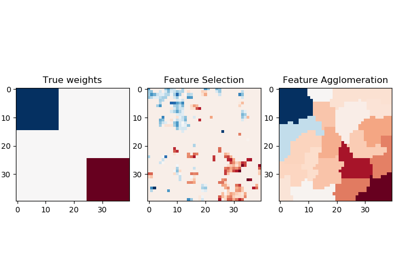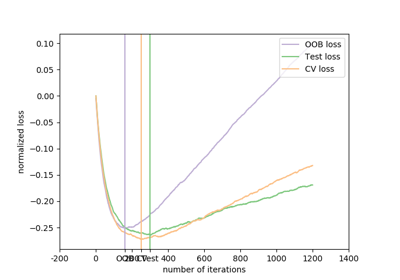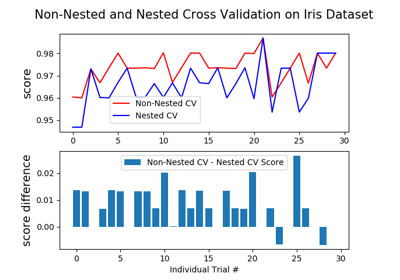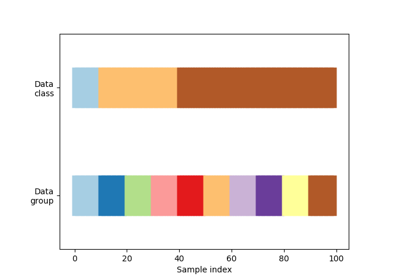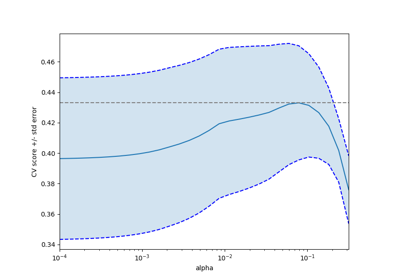sklearn.model_selection.KFold¶

class sklearn.model_selection.KFold(n_splits=5, shuffle=False, random_state=None)[source]

K-Folds cross-validator

Provides train/test indices to split data in train/test sets. Split dataset into k consecutive folds (without shuffling by default).

Each fold is then used once as a validation while the k - 1 remaining folds form the training set.

Read more in the User Guide.

Parameters
n_splitsint, default=5

Number of folds. Must be at least 2.

Changed in version 0.22: n_splits default value changed from 3 to 5.

shuffleboolean, optional

Whether to shuffle the data before splitting into batches.

random_stateint, RandomState instance or None, optional, default=None

If int, random_state is the seed used by the random number generator; If RandomState instance, random_state is the random number generator; If None, the random number generator is the RandomState instance used by np.random. Only used when shuffle is True. This should be left to None if shuffle is False.

StratifiedKFold

Takes group information into account to avoid building folds with imbalanced class distributions (for binary or multiclass classification tasks).

GroupKFold

K-fold iterator variant with non-overlapping groups.

RepeatedKFold

Repeats K-Fold n times.

Notes

The first n_samples % n_splits folds have size n_samples // n_splits + 1, other folds have size n_samples // n_splits, where n_samples is the number of samples.

Randomized CV splitters may return different results for each call of split. You can make the results identical by setting random_state to an integer.

Examples

>>> import numpy as np
>>> from sklearn.model_selection import KFold
>>> X = np.array([[1, 2], [3, 4], [1, 2], [3, 4]])
>>> y = np.array([1, 2, 3, 4])
>>> kf = KFold(n_splits=2)
>>> kf.get_n_splits(X)
2
>>> print(kf)
KFold(n_splits=2, random_state=None, shuffle=False)
>>> for train_index, test_index in kf.split(X):
...     print("TRAIN:", train_index, "TEST:", test_index)
...     X_train, X_test = X[train_index], X[test_index]
...     y_train, y_test = y[train_index], y[test_index]
TRAIN: [2 3] TEST: [0 1]
TRAIN: [0 1] TEST: [2 3]

Methods

 get_n_splits(self[, X, y, groups]) Returns the number of splitting iterations in the cross-validator split(self, X[, y, groups]) Generate indices to split data into training and test set.
__init__(self, n_splits=5, shuffle=False, random_state=None)[source]

Initialize self. See help(type(self)) for accurate signature.

get_n_splits(self, X=None, y=None, groups=None)[source]

Returns the number of splitting iterations in the cross-validator

Parameters
Xobject

Always ignored, exists for compatibility.

yobject

Always ignored, exists for compatibility.

groupsobject

Always ignored, exists for compatibility.

Returns
n_splitsint

Returns the number of splitting iterations in the cross-validator.

split(self, X, y=None, groups=None)[source]

Generate indices to split data into training and test set.

Parameters
Xarray-like, shape (n_samples, n_features)

Training data, where n_samples is the number of samples and n_features is the number of features.

yarray-like, shape (n_samples,)

The target variable for supervised learning problems.

groupsarray-like, with shape (n_samples,), optional

Group labels for the samples used while splitting the dataset into train/test set.

Yields
trainndarray

The training set indices for that split.

testndarray

The testing set indices for that split.

Examples using sklearn.model_selection.KFold¶